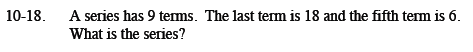Home > CCA2 > Chapter 10 > Lesson 10.1.1 > Problem10-18

10-18.

A series has 9 terms. The last term is 18, and the fifth term is 6. What is the series? Homework Help ✎Find the sequence that corresponds to the terms of the series.

t(5) = 6 = (5)a + b
t(9) = 18 = (9)a + b

a = −3 and b = −9

Now use the sequence to find the rest of the terms of the series.

(−6) + (−3) + 0 + 3 + 6 + 9 + 12 + 15 + 18